# Inequality of the means

 Importance: High ✭✭✭
 Author(s):
 Subject: Geometry
 Keywords: arithmetic mean geometric mean Inequality packing
 Posted by: mdevos on: April 6th, 2009
Question   Is is possible to packrectangular-dimensional boxes each of which has side lengthsinside an-dimensional cube with side length?

Taking the arithmetic/geometric mean inequalitymultiplying both sides byand then raising both sides to thepower yields: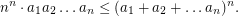So, in the above question, the volume of the cube is at least the sum of the volumes of the rectangular boxes. Furthermore, a positive solution to this question would yield a strengthening of the arithmetic/geometric mean inequality.

For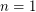the problem is trivial, for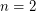it is immediate, and for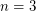it is tricky, but possible. It is also known that a solution for dimensionsandcan be combined to yield a solution for dimension. Thus, the question has a positive answer wheneverhas the form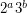. It is open for all other values.

See Bar-Natan's page for more.

## Bibliography

[BCG] E. R. Berlekamp, J. H. Conway and R. K. Guy, Winning Ways for Your Mathematical Plays, Academic Press, New York 1983.

* indicates original appearance(s) of problem.